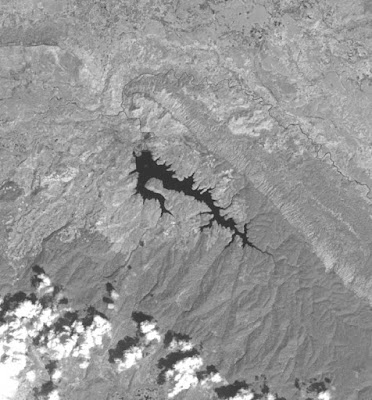## Sunday, November 29, 2015

### Raster math in GRASS GIS using numpy

Houay Lamphan Gnai, yet another hydropower plant just started commercial operations recently with a reservoir area of seven square kilometers as reported by local media. Time to put it on OpenStreetMap using up-to-date Landsat 8 imagery and the new GRASS GIS 7 plugin for QGIS 2.12. To get familiar with GRASS GIS' interface to numpy, I've decided to extract the reservoir using numpy.Houay Lamphan Gnai on Landsat 8 image taken on the 23rd Oct, 2015

As with Landsat 7 I used the near infrared (NIR) channel of Landsat 8 with a wavelength of 0.85 - 0.88 micrometers. Whereas the infrared channel was band 4 in Landsat 7, it is now band 5 in Landsat 8.
The following script extracts pixels representing waterbodies (low values) and creates a new raster layer with 1.0 and null values. It takes as arguments the name of the input map, name of the output map and a threshold value.
```#!/usr/bin/env python

from grass.script import array as garray
import numpy as np
import sys

def main(argv=None):
if argv is None:
argv = sys.argv

inputname = argv
outputname = argv
threshold = np.float_(argv)

in_arr = garray.array()
out_arr = garray.array()
out_arr[...] = in_arr
out_arr[out_arr > threshold] = np.nan
out_arr[out_arr <= threshold] = np.float_(1.0)
out_arr.write(outputname, overwrite=True)

return 0

if __name__ == '__main__':
sys.exit(main())
```
After extracting the reservoir as raster layer, I continued with the previously described workflow.Final result after extracting pixels representing water bodies, growing by one pixel, vectorizing and smoothing

I was wondering about the performance of this script against GRASS GIS' own map calculator. So I compared above script with this one:
```#!/usr/bin/env python

from grass.script.raster import mapcalc
import sys

def main(argv=None):
if argv is None:
argv = sys.argv

inputname = argv
outputname = argv
threshold = float(argv)

expr = '"%s" = if("%s" <= %f, 1, null())' % (outputname,inputname,threshold)
mapcalc(expr, overwrite=True)

return 0

if __name__ == '__main__':
sys.exit(main())
```
For a region with 320223 cells I got the following results:
```script with numpy: 0.357898100217
script with r.mapcalc: 0.270698928833
```
As actually expected r.mapcalc performs better in this simple case. But still the interface to numpy unlocks the great potential and functionality of numpy which excels GRASS GIS' map calculator.Editing the newly created reservoir in JOSM

Is there any other map or dataset than OpenStreetMap that contains this reservoir right now? I don't think so!

#### 1 comment:

1.Awesome & very helpful. Thanks for sharing!